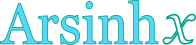## Inverse hyperbolic sine calculator

This tool evaluates the inverse hyperbolic sine of a number: arsinh(x). Enter the argument x below.

 x = Result: arsinh(x) =## Definitions

### General

The inverse hyperbolic sine function, in modern notation written as arsinh(t) or arcsinh(t) or sinh-1t, gives the value x (hyperbolic angle), so that:

The inverse hyperbolic sine function accepts arguments from the whole real space. Since the hyperbolic sine is defined through the natural exponential function , its inverse can be defined through the natural logarithm function, using the following formula, for any real x:

### Properties

The derivative of the inverse hyperbolic sine function is:

The integral of the inverse hyperbolic sine function is given by: